## Example Questions

### Example Question #1 : How To Find The Surface Area Of A Prism

David wants to paint the walls in his bedroom. The floor is covered by a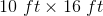carpet.  The ceiling is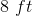tall. He selects a paint that will cover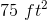per quart and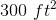per gallon. How much paint should he buy?

3 quarts

1 gallon and 1 quart

1 gallon and 2 quarts

2 gallons and 1 quart

1 gallon

1 gallon and 2 quarts

Explanation:

Find the surface area of the walls: SAwalls = 2lh + 2wh, where the height is 8 ft, the width is 10 ft, and the length is 16 ft.

This gives a total surface area of 416 ft2. One gallon covers 300 ft2, and each quart covers 75 ft2, so we need 1 gallon and 2 quarts of paint to cover the walls.

### Example Question #1 : How To Find The Surface Area Of A Prism

A box is 5 inches long, 5 inches wide, and 4 inches tall. What is the surface area of the box?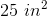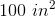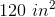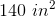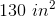Explanation:

The box will have six total faces: an identical "top and bottom," and identical "left and right," and an identical "front and back." The total surface area will be the sum of these faces.

Since the six faces consider of three sets of pairs, we can set up the equation as: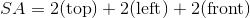Each of these faces will correspond to one pair of dimensions. Multiply the pair to get the area of the face.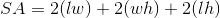Substitute the values from the question to solve.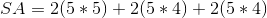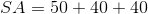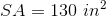### Example Question #7 : How To Find The Surface Area Of A Prism

What is the surface area of a rectangular brick with a length of 12 in, a width of 8 in, and a height of 6 in?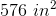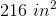None of the answers are correct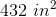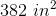Explanation:

The formula for the surface area of a rectangular prism is given by:

SA = 2LW + 2WH + 2HL

SA = 2(12 * 8) + 2(8 * 6) + 2(6 * 12)

SA = 2(96) + 2(48) + 2(72)

SA = 192 + 96 + 144

SA = 432 in2

216 in2  is the wrong answer because it is off by a factor of 2

576 in3 is actually the volume, V = L * W * H